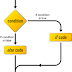This is used to get a decision in programming language. There are four basic types of "If statements". First we look at the structures of them and then try programs.

Structure 01

```if ( condition )
{
//statement executes when boolean condition is true
}
```

```public class Apple {
public static void main(String[] args) {

int x = 12;

if (x == 10) {
System.out.print("X: " + x);
}
}
}```

Structure 02

```if ( condition )
{
//statement executes when boolean condition is true
}
else
{
//statement executes when boolean condition is false
}

```

```public class Apple {
public static void main(String[] args) {

int x = 12;

if (x == 10) {
System.out.print("X: " + x);
} else {
System.out.print("X is invalid ");
}
}
}

```

Structure 03

```if ( condition one )
{
//statement executes when boolean condition one is true
}
else if(condition two )
{
//statement executes when boolean condition two is true
}
else if(condition three )
{
//statement executes when boolean condition three is true
}
else
{
//statement executes when all boolean conditions are false
}

```

```public class Apple {

public static void main(String args[]) {

int x = 85;

if (x < 35) {
}

else if (x < 55) {
}

else if (x < 65) {
}

else if (x < 75) {
}

else {
}

}
}

```

Structure 04

```if( condition X )
{
if(condition Y)
{
//statement executes when boolean condition Y is true
}
else
{
//statement executes when boolean condition Y is false
}
}
else
{
//statement executes when boolean condition X is false
}

```

```public class Apple {
public static void main(String[] args) {

int x = 10;

if (x == 10) {
if (x < 5) {
System.out.print("x < 5");
} else {
System.out.print("x > 5");
}
} else {
System.out.print("X is invalid ");
}
}
}

```

You can use if-else without curly brackets.

```public class Apple {

public static void main(String args[]) {

int x = 20;

if (x != 10)
System.out.println("X is not equal to 10");

/*
* if you don't add "{}" it will automatically add the brackets and
* close the bracket after the first execution line
*/

}
}

```

```public class Apple {

public static void main(String args[]) {

int x = 20;

if (x != 10)
System.out.println("X is not equal to 10");
/*
* if you don't add "{}" it will automatically add the brackets and
* close the bracket after the first execution line
*/
}
}

```

```public class Apple {

public static void main(String args[]) {

int x = 20;

if (x != 10)
;
else
;
System.out.println("x == 10");

/* This is legal but you can't add any other line between if and else */
}
}

```

If statementReviewed by Ravi Yasas on 1:39 AM Rating: 5